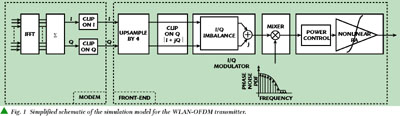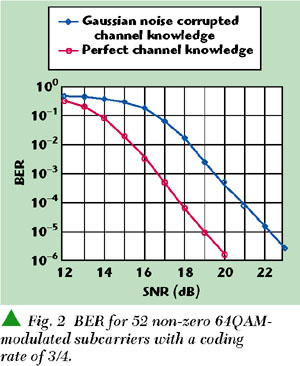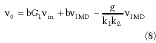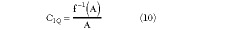This article describes origins of the use of high electron mobility field-effect transistors (HEMT) based on AlGaN/GaN technology as control components for high power microwave and RF control applications.

B. Côme, R. Ness, S. Donnay,
L. Van der Perre, W. Eberle,
P. Wambacq, M. Engels
and I. Bolsens
IMEC v.z.w., DESICS
Heverlee, Belgium

New orthogonal frequency-division multiplex (OFDM)-based wireless local area network (WLAN) standards1,2 will allow local networks to go wireless, while offering multimedia communications in the 5 GHz band for consumer multimedia applications. The basic principle of OFDM is to split a high rate datastream into Nc lower rate streams that are transmitted simultaneously over Nc subcarriers. As a result, because the symbol duration increases on each parallel subcarrier, the relative amount of dispersion in time caused by multipath delay spread is decreased.8 Equivalently, formulated from a frequency domain point of view, the available bandwidth W is divided into a number Nc of subbands of width W/Nc , and the high rate datastream is transmitted in parallel by modulating Nc carriers at a rate Nc times lower than the data symbol rate R. If the bandwidth of each subband is sufficiently small compared to the coherence bandwidth of the channel (W/Nc >> Bcoh ), each subband experiences flat fading, which makes equalization much simpler. Furthermore, intersymbol interference (ISI) can be eliminated by introducing a guard interval every OFDM symbol, and intercarrier interference (ICI) can be avoided with a cyclic extension of each OFDM symbol. As a result, prepending each OFDM symbol with a cyclic prefix (a copy of the last Ng samples of the OFDM symbol) eliminates completely ISI and ICI, provided that Ng is large enough.

Digital OFDM transceiver ICs have already been presented3 ; it is now a challenge to build integrated front-ends with minimum degradation of the overall performance demonstrated at baseband by such modems. Few studies have been published that quantify the front-end effects on the link performances in terms of implementation loss (additional signal-to-noise ratio (SNR) required to achieve a given bit error rate (BER) when a front-end effect is considered) and provide specifications to analog front-end designers. Given the high data rates with required low BERs, and given the nature of the OFDM signal, a conservative analysis of the front-end requirements leads to severe, over-dimensioned specifications. Such a design would never meet the market for consumer applications, which is by necessity both low cost and low power.

To extract more optimal front-end specifications, each design choice is assessed from a system point of view, through the evaluation the BER performance of the complete WLAN-OFDM link, including the digital modem and all its functionalities, the mixed signal front-end and the channel. This article describes the simulation model and shows results for the effect of digital clipping in the transmit modem and the influence of the transmit symbol wordlength. Finally, more effects are presented related to the implementation loss (IL) introduced by the analog front-end -- effects on the BER of a nonlinear power amplifier, phase noise and finally I/Q imbalance.

WLAN-OFDM LINK MODEL AND BASEBAND SIMULATION SETUP

For this study, the complete WLAN-OFDM link was modeled in MATLAB, comprising models for a digital baseband OFDM modem, a generic front-end and a set of multipath channels corresponding to an indoor environment (of which the frequency response was determined by ray-tracing simulation4 ).

The principle of OFDM is the parallel transmission of quadrature amplitude modulation (QAM)-modulated subcarriers using frequency division multiplexing. The time domain symbols are generated from the modulated subcarriers through an inverse fast Fourier transform (IFFT), then I/Q modulated, upconverted to the desired frequency band with the adequate power to be transmitted through the channel. At the receive side, they undergo the inverse operations. Figure 1 shows a simplified schematic of the transmitter simulation model in our simulation setup.OFDM systems with 52 non-zero 64QAM-modulated carriers1,2 produce time-domain signals with a crest factor of 19, which would lead to very inefficient implementations in terms of wordlength in the digital modem and data converters, and of dynamic range in the analog front-end. Therefore, signals coming out of the IFFT should be clipped to reduce large crest factors inherent to digital multiplexed trunk (DMT) modulation techniques. This operation is performed digitally in the modem, right after the time-domain signal generation.

The spectrum of the OFDM signal1,2 occupies 16.8 MHz in a 20 MHz channel, and the digital modem produces I/Q signals sampled at 20 MHz. To avoid high sample rates when simulating this bandpass signal at 5.2 GHz, simulations are conducted at baseband (BB) using its complex low pass equivalent representation. Yet, simulation oversampling by 4 is added in the interface between the modem and the front-end so that nonlinear distortion can be evidenced (in this study, up to an order 3). This over-sampling can also be present as a hardware implementation, in the case where the I/Q modulation is performed digitally. It will also be shown that introducing further clipping on the modulus of the upsampled signal helps improve the system power efficiency.

Finally the analog front-end model consists of three blocks: an I/Q modulator adding I/Q imbalance; a mixer with a local oscillator signal defined by its phase noise power density function; and power amplifier (PA) exhibiting a cubic nonlinearity (an ideal power control module keeps the PA average RF input power constant to make fair comparisons between transfer functions).

These effects are considered at the transmit side only. Nonetheless, simulations show that they produce similar BER degradation if placed at the receive side, even when considering multipath channels.

Perfect/Corrupted Channel Knowledge

Channel equalization is performed at the receiver on the basis of the received long training symbol (LTS), which is a predefined OFDM-symbol prepended to the transmitted payload. At the receive side, the equalizer estimates the channel coefficients from the received LTS. Perfect channel knowledge is assumed when the LTS is kept noise-and-distortion free. On the contrary, if the thermal noise from the channel (white in a Gaussian channel, colored in multipath channels) as well as front-end nonidealities corrupt the payload as well as the LTS, then the channel estimation is impaired and the channel equalization is imperfect. The current model used for the equalizer corresponds to a kind of worst-case implementation, as none of these corruptive effects is compensated for; it typically results in an additional IL of 3 dB at BER = 10­5 . Figure 2 shows BER curves for 52 non-zero 64QAM modulated subcarriers with a coding rate of 3/4, showing a 3 dB implementation loss due to corruption of the long training symbol not corrected in the equalizer.All the simulation results presented -- unless stated otherwise -- are for 52 non-zero 64QAM-modulated subcarriers, with a coding rate of 3/4 (the most demanding case in1,2 ) and imperfect channel knowledge. As non-ideality effects are more visible in a Gaussian channel than in a multipath channel, only Gaussian channels are considered in this article.

Validity of the Model

This model must be validated by comparing theoretical BER curves and BER curves resulting from simulations. Such a comparison is illustrated here for uncoded and coded BPSK transmission.

Bit error probability PB for uncoded BPSK9 is

PB = Q(2Eb /No )

where

Eb /No    = W/R * SNR * k (1)

Q(x) = 1/2* erfc(x2), where erfc(x) is the MATLAB built-in comlementary error function

W = 16.6 MHz is the bandwidth of the modulated signal

R = 12 Mbps is the uncoded BPSK data rate

k = 64/(16+64) is a correcting factor because part of the energy is lost in the guard interval added in front of each OFDM symbol

ThereforeFigure 3 shows the theoretical BER curve PB versus SNR for uncoded BPSK plotted together with the BER curve resulting from simulation. The WLAN-OFDM link model used for this simulation was implemented with upsampling by 4 at Tx and downsampling by 4 at Rx, plus all other non-idealities in the Tx and Rx front-end as described previously, but set to negligible values. Furthermore, perfect equalization at the receiver is assumed.The two curves match by 0.15 dB in SNR at BER = 10­4 and by less than 0.05 dB in SNR at BER = 10­5 . The result validates the models in this system simulation tool.

WORDLENGTH OF THE TRANSMITTED SYMBOLS AND OPTIMAL CLIPPING LEVEL

The wordlength b of the symbol at the output of the transmit digital modem has a major impact both on implementation cost and performance limitation. As b decreases, the power consumption and complexity of the digital-to-analog and analog-to-digital converters (DAC/ADC) decreases at the expense of the quantization noise -- hence the BER performance.

However, b can be limited with acceptable performance degradation, due to the limited SNR on the channel. Furthermore, the digital clipping operation after the IFFT limits the crest-factor of the signal, enhancing for a given b the average-signal/quantization-noise power ratio. Still, it also introduces an additional noise source: clipping noise. Therefore, it is a joint-optimization process on b and the normalized clipping level µ (ratio of the clipping level to the rms amplitude δ of the time-domain signal).

For a given wordlength b, an optimal clipping level can be derived5 , since it is a trade-off between two noise sources -- lowering the clipping level enhances the clipping noise while at the same time reducing the quantization noise.

Figure 4 shows the SNR of an OFDM signal after clipping and quantizing plotted versus µ for wordlengths b ranging from 6 to 9 bits: digital clipping at 4is close to the optimal clipping level for all wordlengths. Hence, b can now be optimized separately.Figure 5 shows results from BER simulations (in this particular case, uncoded) considering only the digital modem and a Gaussian channel -- the IL due to the finite wordlength b is less than 0.2 dB at BER = 10­5 for b ≥ 8. Taking into account implementation cost, it is concluded that an 8-bit wordlength with digital clipping at 4is optimum. Extensive simulations were performed using multipath channels to check the crest factor regrowth due to nonlinear effects. As it turns out, it never exceeded 150 percent. This results in the specifications of 8-bit DACs of which the 2 MSB are for signal amplitudes aboveand 10-bit ADCs (2 more bits to accommodate for crest factor regrowth due to nonlinear effects and imperfect AGC at the receiver).INFLUENCE OF THE POWER AMPLIFIER (PA) NONLINEARITY

A linear PA is required due to non-constant envelope signals. The PA is assumed class A with back-off.

The linearity of the PA is a key parameter as it is closely related to power consumption and to distortion, hence BER. The PA is quantified by its input-referred third-order intercept point IIP3 or 1 dB-compression point IP1dB for a given average RF input power PIN .

PA Amplitude Transfer Function Model

The nonlinear amplitude transfer function of a PA with a linear gain G, assuming that the PA is kept out of saturation, was modeled by a cubic nonlinearity such that

y = G(x­x3 ) for x < xsat (3)

where

xsat          = limit of the saturation region

The nonlinearity coefficient * can be expressed as a function of IIP3, IP1dB , or xsat asBecause real life systems do not present only cubic nonlinearity, IIP3 and IP1dB are generally not related by the well-known equation IIP3 = IP1dB + 9.6 dB, but rather IIP3 > IP1dB + 9.6 dB. Therefore, it is best to characterize the nonlinearity of the PA by its IP1dB to make sure that the average signal power is far enough from the PA compression region. However, as in-band distortion will be introduced mainly by cubic nonlinearities, the distortion created by other orders can be filtered out and eliminated. As a result, the specifications derived for cubic nonlinearities only are still valid for PAs with a more complex nonlinear behavior.

PA Model for In-band Distortion

The PA model based on the amplitude transfer function previously described cannot be used as such with a low pass representation of the signal, as at BB only in-band distortion may be computed. Instead, the model for BB simulation is based on two functions, the AM-AM and AM-PM conversion functions, f and g, respectively.

If

x(t) = A(t)ejΦ(t) (5)

then

y(t) = f[A(t)]ej(Φ(t)+g[A(t)]) (6)

From Equation 1Therefore, the PA model for BB simulation, computing in-band distortion only isNote that as f(t) and g(t) are functions of |x(t)| only, the PA exhibits envelope (bandpass) nonlinearity. This allows the PA to be linearized with predistortion techniques.

To enforce the condition that the PA remains out of saturation even if (IP1dB ­ PIN ) < +8.4 dB, as shown in Figure 6, a lower clipping level µ* would be required. However, it was shown that as far as clipping at BB is concerned, µ = 4 is optimal. A possible solution is to introduce additional digital clipping after the upsample by 4. As the additional clipping noise is not confined in-band anymore, it introduces lower SNR degradation, and the signal can still be clipped to xsat. Moreover, this operation is performed on the modulus of the signal and not on I and Q separately. This is done at the expense of the computational complexity. It does, however, improve the BER performance.Predistortion with Look-up Table

Digital predistortion can be added to the signal so that the PA amplitude transfer function is linearized. It has been shown that for an input signal x(t) = A(t)ejΦ(t) , the output y(t) of the PA can be written as (for A ¾ xsat )

y(t) = f[A(t)]ejΦ(t) (9)

where

f(A) = AM-AM conversion function of the PA

Therefore, the PA can be linearized by predistorting A as f­1 (A).

The operating principle of the linearization technique implemented in this model is shown in Figure 7:10 after the upsample by 4 function, the modulus of I + jQ is computed and passed first to the clip on |I+jQ| block. It then addresses a look-up table that returns the predistortion factor CIQ that weights both I and Q. CIQ is given bywhere

f­1 = inverse function of the AM-AM conversion function

Therefore, f(A) should be measured or estimated for the selected PA (or type of PA) and the coefficients CIQ estimated and stored in a look-up table, for all A ¾ xsat .

The signal at the output of the PA then is

y(t) = f(CIQ |I+jQ|)ejΦ(t) (11)

and

f(CIQ |I+jQ|) = f(f­1 (A)) = min{GA; G(xsat ­xsat 3 )}

then

y(t) = min{Gx(t); G(xsat ­xsat 3 )ejΦ(t) } (12)

The operation of predistortion on the input and output signals of the front-end is plotted. For simplicity, the linear gain of the PA is 1, all the signals have a 8-bit wordlength (hence represented by integers between ­128 an +127), and the I and Q signals are first clipped at 4in the digital modem, and then upsampled by 4 in the mixed-signal front-end. Finally the modulus of I + jQ is clipped to 2.81to reduce the back-off in the PA, which is such that (IP1dB ­ PIN ) is 5.4 dB. Figure 8 shows the data plot.The effects introduced by the limited wordlength of I, Q and CIQ are not visible on this large scale figure. However, the amplitude difference between the input signal after the second clipping operation and the linearized output signal is less that 0.5 LSB up to the point where the PA in-band output signal reaches its saturation level. Note that the crest factor at the output of the PA has been reduced from 2.81* to 2.46* due to the gain compression of the PA.

BER Simulations Results for PA Nonlinearity

Figure 9 shows that for low IL, the PA (without predistortion) can be operated with only 5.4 dB back-off between PIN and IP1dB (15 dB between PIN and IIP3). (The IL at BER = 2.10­5 is 1.9 dB for (IP1dB ­ PIN ) = 4.4 dB, 0.5 dB for (IP1dB ­ PIN ) = 5.4 dB.) This result can be translated in terms of system power efficiency *, assuming a PA intrinsic power efficiency of 50 percent:= 13 percent. The BER degradation is mainly introduced by the additional clipping, and not by the gain compression in itself. With ideal predistortion applied as a linearization technique, the BER performance did not improve much. Thus, linearizing the PA by predistortion will not help significantly with respect to the system power efficiency/BER trade-off. However, it might be considered to reduce out-of-band radiated power (intermodulation products and harmonics).INFLUENCE OF PHASE NOISE

The degradation of a multi-carrier OFDM signal because of oscillator phase noise results from two kinds of effects. The first one is a close-in phase error common to all subcarriers, which rotates the entire constellation. This error can be estimated and compensated.6 The second one, which has a higher frequency Gaussian-like noise behavior, cannot be corrected. It results in inter-subcarrier interference.

The SNR (hence the BER performance) that can be achieved in an OFDM system with a noisy VCO can be approximated by integrating its phase noise power density function (PDF) over the channel bandwidth; this approximation does not take into account the fact that the common contribution can be corrected.

In the previously shown setup, the BB OFDM signal is mixed with a BB, time-domain representation of the frequency synthesizer (FS) signal. The phase noise PDF of a FS is approximated by a Lorenzian function with uniform phase distribution. It is parameterized by its total integrated phase noise K and ­3 dB bandwidth B,7 to which a noise floor L0 is superimposed (set 35 dB above the thermal noise) asIt is then converted to the time-domain by an IFFT.

BER Simulations Results with Phase Noise

The shape of the phase noise PDF has little impact: performances are mainly determined by the total integrated phase noise K. Figure 10 shows that a K value of ­32 dBc results in low BER degradation. With the Lorenzian model and B = 200 kHz, this can be translated into a VCO phase noise specification of ­85 dBc at 100 kHz offset.INFLUENCE OF I/Q IMBALANCE

I/Q imbalance results from two effects: gain mismatch between the I and Q paths and nonperfect quadrature generation (ε and ΔΦ, respectively). I/Q imbalance is often specified in terms of negative frequency rejection (NFR) in dBc; that is, a mismatch between the I and Q paths has the same effect as a parasitic up/downconversion by a tone at the negative frequency of the LO frequency such that

(1+ε)cos(ωc t+ΔΦ)+j(1­*)sin(ωc t+ΔΦ)(1+jεtan(ΔΦ)e+jωct +(ε­jtan(ΔΦ))e­jωct (14)

(ε, ΔΦ) are related to NFR by

NFR ≈ 10log(ε2 +tan2 (ΔΦ)) (15)

I/Q imbalance on x(t) = I + jQ is simulated at BB with

y(t) = (1­ε)e­jΔΦ I(t) + j(1+ε)e+jΔΦ Q(t) (16)

BER Simulations Results with I/Q Imbalance

The following results were extracted from BER curves as previously displayed, for similar coding conditions and in a Gaussian channel, given in terms of NFR at BER = 2.10­5 . For NFR of 32, 29 and 26 dBc, the IL is 0.3 dB, 0.8 dB and 1.9 dB, respectively.

CONCLUSION

It was shown that a WLAN OFDM-based link exhibits acceptable BER performance degradation with an 8-bit wordlength for the transmitted symbols and a crest factor of the transmitted signal digitally limited to 4* at baseband. Some effects of the analog front-end were also investigated. The use of a class A power amplifier with only 5.4 dB back-off between the average input power and the input-referred P1dB (additional signal processing is then required to keep PA out of saturation) was demonstrated. In terms of IL the influence of the frequency synthesizer total integrated phase noise and of I/Q imbalance was quantified.

These results were derived from a simulation setup implemented in MATLAB that assesses the BER performance of a complete WLAN-OFDM link. Such a simulation setup is a powerful tool to gain insight in BER degradation along the complete link due to front-end non-idealities, and to trade-off IL among the different front-end non-idealities on the one hand, and IL in the digital part versus IL in the front-end on the other.

ACKNOWLEDGMENT

This work was partially supported by grants from the Office of Naval Research and the National Science Foundation. The material for this article was first presented at the RAWCON2000 IEEE Radio and Wireless Conference held in Denver, CO in September 2000. *

References

1. IEEE P802.11a/D7.0, Draft Supplement to Standard for LAN/MAN Part 11: MAC&PHY Specifications: High Speed Physical Layer in the 5 GHz Band, July 1999.

2. ETSI <DTS/BRAN-0023003-1> V0.k, Broadband Radio Access Networks; HIPERLAN Type 2 Functional Specification; Part 1 -- Physical (PHY) Layer, October 1999.

3. W. Eberle, M. Badaroglu, et al., "A Digital 80 Mb/s OFDM Transceiver IC for Wireless LAN in the 5 GHz Band," IEEE ISSCC Proceedings, February 2000.

4. P. Vandenameele, L. Van der Perre, et al., "An SDMA Algorithm for High-speed Wireless LAN," Globecom 98, November 1998, pp. 189­194.

5. D.J.G. Mestdagh, P.M.P. Spruyt, et al., "Effect of Amplitude Clipping in DMT-ADSL Transceivers," Electronic Letters, Vol. 29, No. 15, pp. 1354­1355.

6. C. Muschallik, "Influence of RF Oscillators on an OFDM Signal," IEEE Transactions on Consumer Electronics, Vol. 41, No. 3, August 1995, pp. 592­603.

7. A.R.S. Bahai and B.R. Saltzberg, Multi-carrier Digital Communications, Theory and Application of OFDM, Kluwer Academic/Plenum Publishers, 1999.

8. R. Van Nee and R. Prasad, OFDM for Wireless Multimedia Communications, Artech House Publishers, 2000.

9. B. Sklar, Digital Communications, Fundamentals and Applications, Prentice-Hall International, 1988.

10. S. Uwano, Y. Matsumoto, et al., "Linearized Constant Peak-power Coded OFDM Transmission for Broadband Wireless Access Systems," IEICE Trans. Commun., Vol. E82-B, No. 12, December 1999.